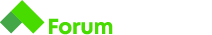# Mock Exam (Variant 1) - Crooked Digits

Здравейте, мога ли да помоля някой да ми обясни две неща:

• от къде идва стойноста “(x => x - 48)” в следното решение

string input = Console.ReadLine();

``````        do
{
var result = (input.ToList().Where(x => Char.IsDigit(x)).
ToList().Sum(x => x - 48).ToString());
input = result;

} while (input.Length > 1);

Console.WriteLine(input);
``````

Вторият ми въпрос е, ако е формула, защо я няма в обучението и ако пък все пак я има къде е " C# Fundamentals"
Заповядайте и условието
" Crooked Digits

The crooked digit of a given number N is calculated using the number’s digits in a very weird and bendy algorithm. The algorithm takes the following steps:

1. Sums the digits of the number N and stores the result back in N.
2. If the obtained result is bigger than 9 , step 1 . is repeated, otherwise the algorithm finishes.

The last obtained value of N is the result, calculated by the algorithm.

# Input

• The input data should be read from the console.
• The only line in the input contains a number N, which can be an integer or real number (decimal fraction), positive or negative.
• The input data will always be valid and in the format described. There is no need to check it explicitly.

# Output

• The output data should be printed on the console.
• You must print the calculated crooked digit of the number N on the first and only line of the output.

# Constraints

• The number N will have no more than 300 digits before and after the decimal point.
• The decimal separator will always be the “.” symbol.

# Sample tests

## Input

``````3
``````

## Output

``````3
``````

Благодаря!

Здравей и добре дошъл, @boreto,

Ще предположа, че това решение не е твое, а си го получил без допълнителен контекст или разясняване.

Най-общо казано, компютрите разбират единствено от цифри, което значи, че зад всеки знак или character стои някаква цифрова репрезентация. Например, знак за удивителен ‘!’ има стойността 33, докато знак за събиране ‘+’ има стойност 43. Тук конкретно е използвана стойността 48, защото от 48 започват самите числа - зад стойност 48 се крие числото 0, зад 49 стои 1 и т.н. до 57, където е 9. Всичко това е според един стандарт наречен ASCII - American Standard Code for Information Interchange. За него повече тук.

Обратно към самата задача - както вече може би се досещаш решението, което си ни показал включва неща, които не са разглеждани в подготвителните материали, а именно ASCII стойности, както и LINQ extension методи(Where, ToList, Sum). Знания по тези теми не са необходими за решаването на тази или пък която и да е другa задача от подготвителните материали, но разбира се подобна информация би помогнала за едно по-различно решение от това, което се очаква.

Поздрави,
Радко

2 Likes

Благодаря, за подробния отговор, което дава решение на много мои въпросителни. Между другото тази таблица ,я има в подготвителните материали.

Благодаря!